# Revolutionary, Animal inspired and Challenging Algorithms with interesting GIFs

Over the years, various discoveries of ingenious computer algorithms revolutionized the field of computation.  Computer programming is a vast field with a variety of distinctive pleasures associated with it. These functions have been used to manufacture diversely intricate computer programs that are major contributors for the advancement of current computer age.

## Algorithms that Revolutionized Computing

### 1) Huffman Coding

The Huffman coding algorithm is widely used in lossless data compression methods. Due to the simplicity and rapidness, the algorithm is being used in a variety of compression techniques like DEFLATE (PKZIP’s algorithm) and multimedia codecs which include JPEG and MP3.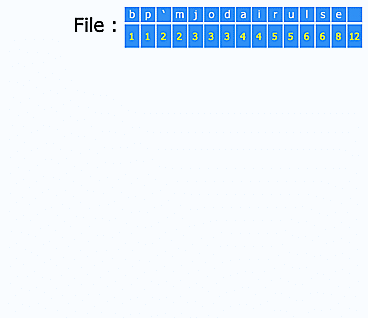### 2) Dijkstra’s Algorithm

This search based algorithm is designed for graphs and solves the single-source shortest path problem for a graph, hence producing the shortest path tree. Using this algorithm, a shortest path on a single map (i.e graph) can be easily determined as shown here.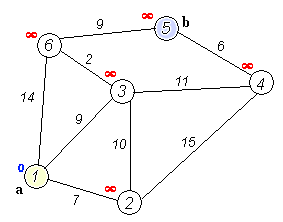### 3) Quick Sort

The algorithm gained its fame when it was implemented in Unix as the default library sort function where it gave its name to the C standard library function as “qsort”.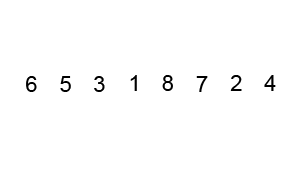### 4) Binary Search Algorithm

A binary search algorithm is used to locate keyword within an array sorted by key value. Sorted lists are usually found in a dictionary where the definition of words is to be found. A telephone book also contains a sorted list of people’s names, addresses, and telephone numbers. One can quickly find a telephone number and address by knowing the desired name.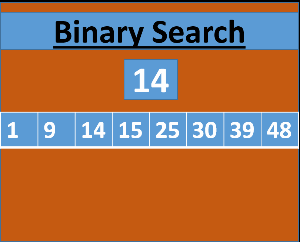### 5) Karatsuba Algorithm

This algorithm is implemented to achieve faster multiplications. Karatsuba Algorithm reduces the digits of numbers to be multiplied and provides a quicker solution.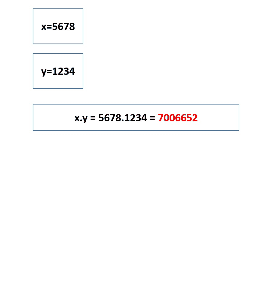### 6) Euclidean Algorithm

Euclidean Algorithm is used to compute the highest common factors, where the largest integer for two positive integers, divides both of them without leaving a remainder. Since it only uses subtraction and comparison to find the HCF, it is invariably used in many advanced algorithms.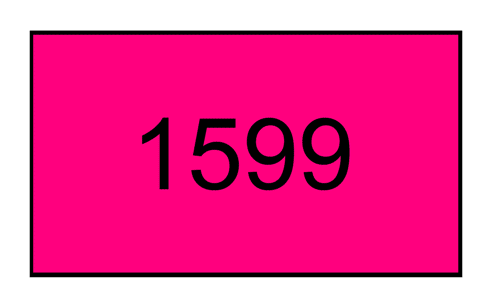## Algorithms that Changed the Course of Computation

### 1) Google PageRank

The PageRank algorithm is one of the very first algorithms developed by Google and is the most renowned algorithm of the current age. PageRank algorithm works by analyzing the links and assigning a numerical weightage to each element of a hyperlinked set of documents where a hyperlink to a page counts as a vote of support, such as the World Wide Web. This helps in measuring the relative importance of the pages within a set. Ultimately a page that is linked to by many pages with high PageRank gains a high rank itself.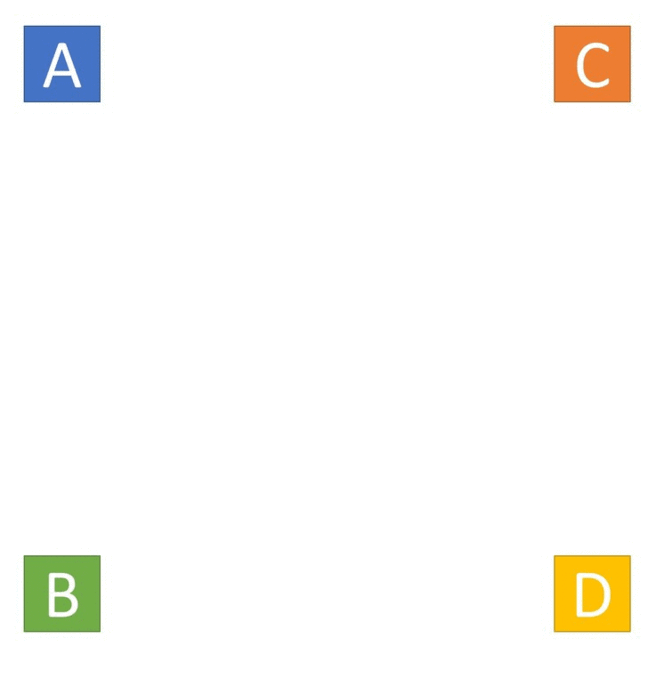### 2) Hamming(7,4)

Hamming(7,4) is a linear error-correcting code. The code is quite effective for transmission of medium situations where burst errors do not occur. In communication, the Hamming code is sued to add three additional parity bits for every four data bits of the message. Hamming’s (7,4) algorithm is then used for the correction of single-bit errors, or the detection of all single-bit and two-bit errors. The received words using Hamming(7,4) can be correctly decoded if they are at most a distance of one from the codeword that was originally transmitted by the sender.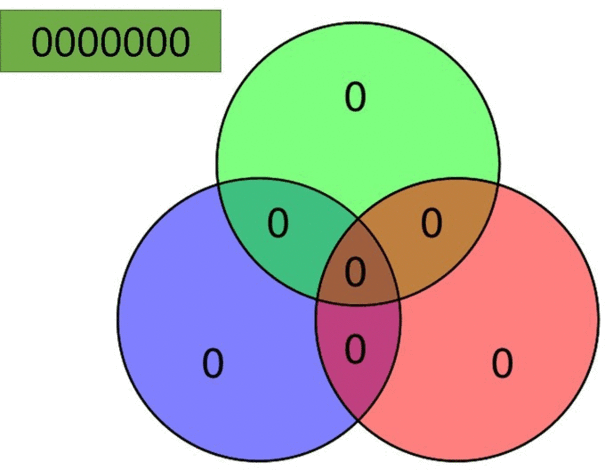## Animal Inspired Algorithms

### 1) Ant Colony Optimization (ACO)

Inspired from Ants, the ACO is an algorithm that is based on probabilistic technique used to solve computational problems. ACO is used to determine reasonably better paths to follow through graphs.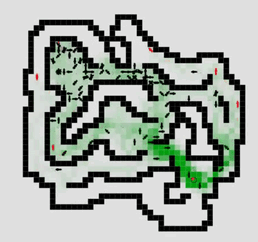### 2) Caterpillar Tree

A Caterpillar Tree is a tree in which all the vertices are within distance 1 of a central path.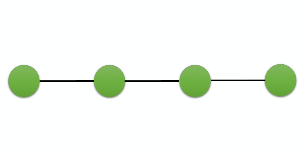## Challenging Algorithms to make you think outside the box

### 1) Towers of Hanoi

It is actually a mathematical puzzle or a game which was publicized for the first time by the French mathematician Édouard Lucas in 1883. The problem consists of three rods and a number of different sized disks that can slide on any rod. Initially the disks are arranged in the ascending order according to the sizes thereby forming a conical shape. The objective of the puzzle is to slide the entire stack from one rod to another with a set of set rules to follow as well. A further modified version of the problem is known as Tower of London which is implemented in the neuropsychological diagnosis and treatment of exclusive functions.### 2) The Dining Philosophers Problem

The Dining Philosophers problem is used in the field of computer science to resolve concurrent algorithm design which involves illustration of synchronization issues and techniques. The problem was formulated by Edsger Dijkstra in 1965 as a student exam exercise.### 3) Traveling Salesman Problem

Traveling Salesman Problem is a special case of Traveling Purchaser Problem. The problem inquires the following query: “Provided a list of cities and the distances between each pair of cities, determine the shortest possible route that passes through each city only once and returns back to the origin city? The problem is specifically an NP-hard problem associated with combinatorial optimization, and has a significant role in theoretical computer science and operations research. The Traveling Salesman Problem has several different applications even without any modifications in its formulation, such as planning, manufacture of microchips and logistics.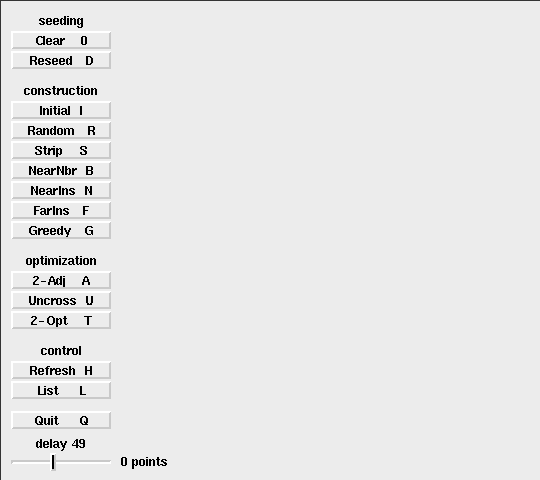17006   12/06/2014